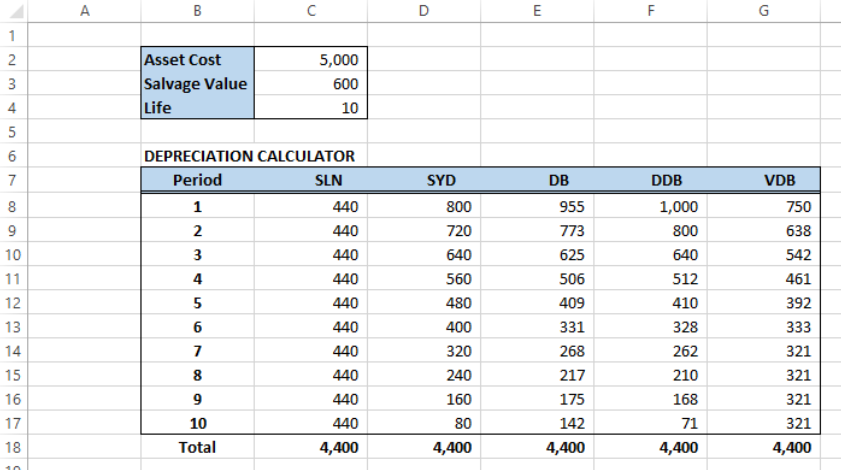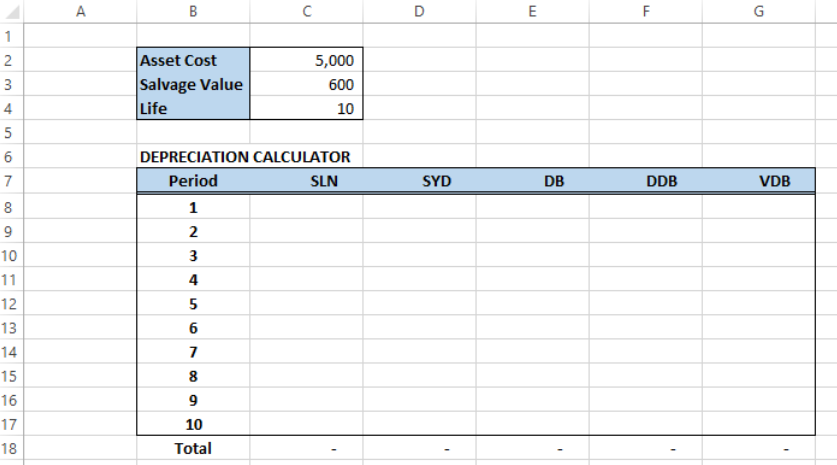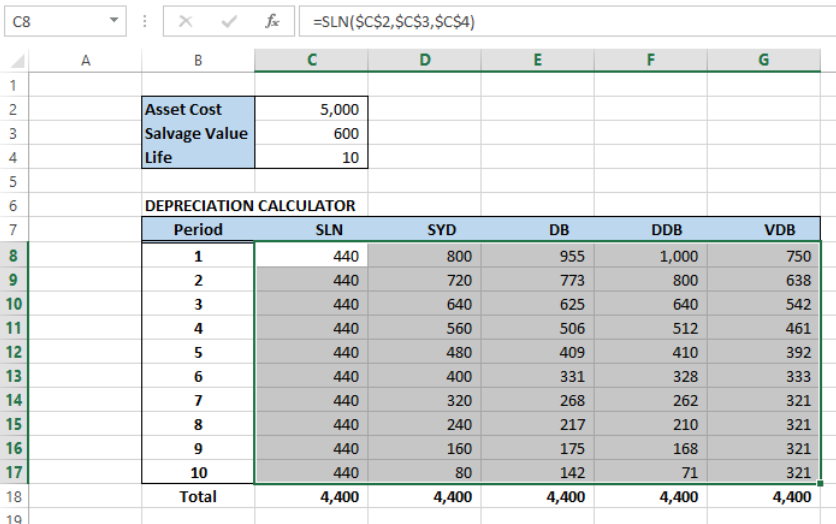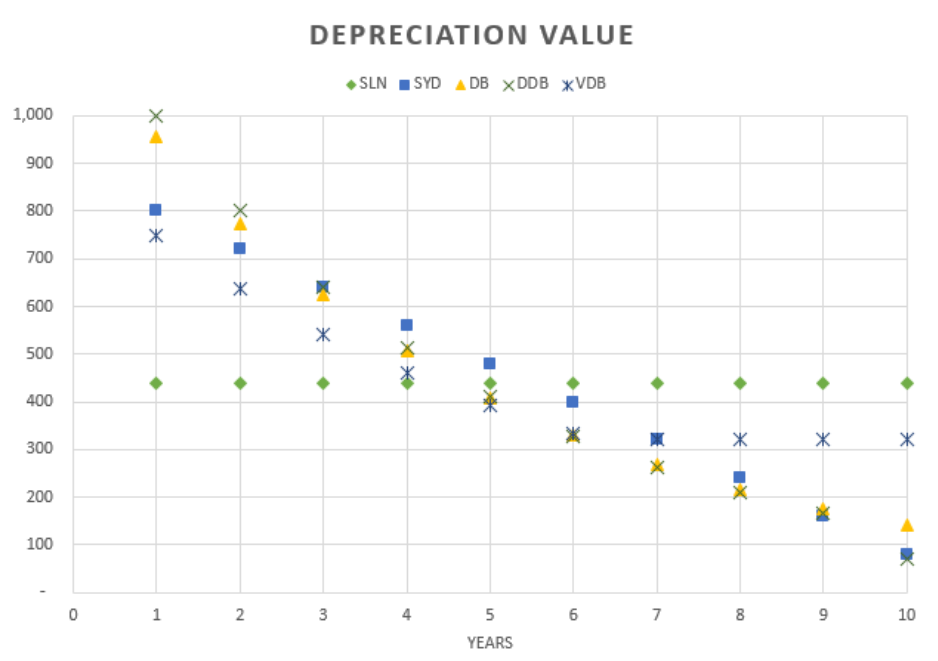Get instant live expert help with Excel or Google Sheets“My Excelchat expert helped me in less than 20 minutes, saving me what would have been 5 hours of work!”

#### Post your problem and you’ll get expert help in seconds.

Your message must be at least 40 characters
Our professional experts are available now. Your privacy is guaranteed.

# Depreciation in Excel – Excelchat

Assets lose value over time. The depreciation expense of an asset is deducted from the net income, and taxes are paid on income after depreciation.  The method of depreciation varies according to an asset’s actual usage or on its projected loss of value.Figure 1.  Final result:  Depreciation formula

There are five types of depreciation methods in Excel.  This article will guide us on how to create a depreciation calculator using:

• SLN Straight-line depreciation method
• SYD – Sum-of-year’s digits depreciation method
• DB – Fixed declining balance depreciation method
• DDB Double declining balance method
• VDB   – Variable declining balance method

For an asset with an initial cost of \$5,000, a salvage value of \$600 and a useful life of 10 years, we want to calculate the depreciation per year up to the tenth year.

## Depreciation calculator

Let us first create a depreciation calculator using the template below:Figure 2.  Depreciation Calculator Template

## Syntax of Depreciation functions

SLN: `=SLN(cost, salvage, life)`

SYD: `=SYD(cost, salvage, life, period)`

DB: `=DDB(cost, salvage, life, period, [factor])`

DDB: `=DDB(cost, salvage, life, period, [factor])`

VDB: `=VDB(cost, salvage, life, start_period, end_period, [factor], [no_switch])`

Parameters:

• Cost – the asset’s initial cost
• Salvage – the asset’s value at the end of depreciation
• Life – the asset’s useful life
• Period – period; has the same unit as life
• Factor – the rate of depreciation of the balance; if omitted, the default value is 2 (refers to double-declining balance method)
• No_switch – optional; if FALSE, function will switch to straight-line depreciation when the depreciation is greater than the calculation for declining balance

## Calculate depreciation

In order to calculate the depreciation using the different methods, we follow these steps:

• Enter the following formulas:

In cell C8: `=SLN(\$C\$2,\$C\$3,\$C\$4)`

In cell D8: `=SYD(\$C\$2,\$C\$3,\$C\$4,B8)`

In cell E8: `=DB(\$C\$2,\$C\$3,\$C\$4,B8)`

In cell F8: `=DDB(\$C\$2,\$C\$3,\$C\$4,B8)`

In cell G8: `=VDB(\$C\$2,\$C\$3,\$C\$4,B8-1,B8,1.5)`

• Select cells C8 to G8
• Press Ctrl + C then drag the fill handle down to row 17.Figure 3.  Output: Depreciation Calculator

We have now calculated the depreciation using the five different methods.  Note that the total depreciation at the end of the useful life of ten years is the same for all methods, \$4,400.

In order to visualize the difference among the methods, we can create a series chart as shown below:Figure 4.  Comparison of Depreciation Methods

## Instant Connection to an Excel Expert

Most of the time, the problem you will need to solve will be more complex than a simple application of a formula or function. If you want to save hours of research and frustration, try our live Excelchat service! Our Excel Experts are available 24/7 to answer any Excel question you may have. We guarantee a connection within 30 seconds and a customized solution within 20 minutes.